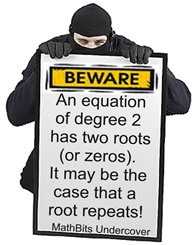Quadratic Formula MathBitsNotebook.com Terms of Use   Contact Person: Donna Roberts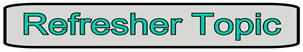Look out! Our math ninjas are back!!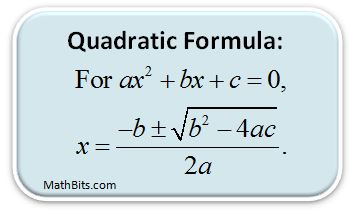The solutions for some quadratic equations are not rational, and cannot be obtained by factoring.
The
quadratic formula, however, may be used to solve ANY quadratic equation (even the ones that can be factored). This is a formula that you want to know and remember!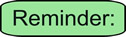• As you can see in the formula, the coefficients (numbers) "a", "b", and "c" from     ax2 + bx + c = 0 are substituted into the formula. • Also notice that the formula will yield two (±) solutions, since a quadratic is a     second degree equation. • The "2a" in the denominator is underneath the entire top, not just the radical. • Be careful with the "signs" of the "a", "b", and "c" values in your calculations.

Let's see the Quadratic Formula at work in various situations: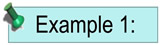Solve: x2 + 2x - 15 = 0 Also factorable. Solution using Quadratic Formula: a = 1; b = 2; c = -15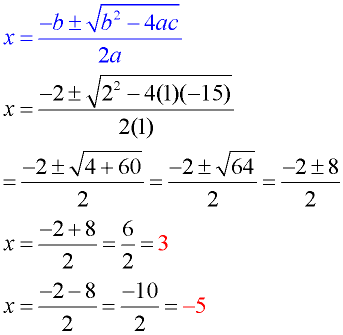This equation is also factorable. (x + 5)(x - 3) = 0 x = -5; x = 3 Notice that the quadratic formula ALSO gives the correct results.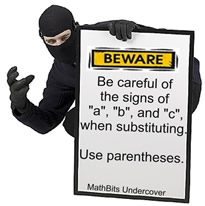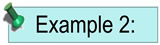Solve: 2x2 - 10x = -3 Not set equal to zero! Set equation equal to zero: 2x2 -10x + 3 = 0 Solution using Quadratic Formula: a = 2; b = -10; c = 3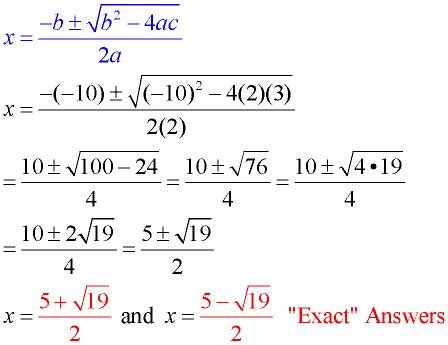Not factorable. Notice the needed parentheses for dealing with the "b" value of "-10".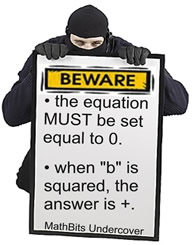Radical answers are"exact" answers. Decimal answers are "approximate" answers.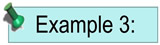Solve: x2 - 6x + 13 = 0 Negative under radical! Solution using Quadratic Formula: a = 1; b = -6; c = 13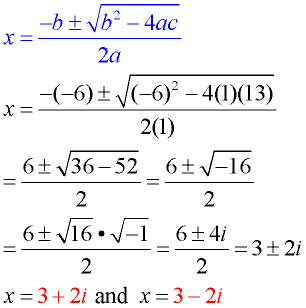Not factorable.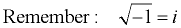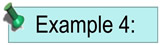Solve: x2 -10x + 25 = 0 Repeated answer! Solution using Quadratic Formula: a = 1; b = -10; c = 25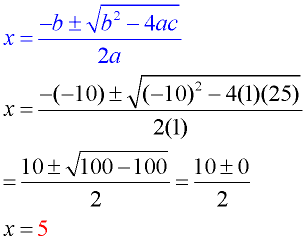Be careful here! It appears that there is only ONE answer, but this is actually a "repeated" root. The graph is tangent to the x-axis at x = 5. This equation is also factorable. (x - 5)(x - 5) = 0 x = 5; x = 5 Answer repeats.